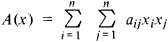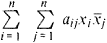(mathematics)
Any second-degree, homogeneous polynomial.
McGraw-Hill Dictionary of Scientific & Technical Terms, 6E, Copyright © 2003 by The McGraw-Hill Companies, Inc.
The following article is from The Great Soviet Encyclopedia (1979). It might be outdated or ideologically biased.

a second-degree form in n variables x1, x2. …, xn that is, a polynomial of these variables, each term of which contains either the square of one of the variables or the product of two different variables. The general form of a quadratic form for n = 2 is

ax12 + bx22 + cx1x2

and for n = 3,

ax12 + bx22 + bc32 + dx1x2 + ex1x3 + fx2x3

where a, b, …, fare any number. An arbitrary quadratic form is written aswhere it is assumed that aij = aji. Quadratic forms in two, three, and four variables are directly connected with the theory of second-order curves (in the plane) and surfaces (in space): in Cartesian coordinates the equation of a centrally symmetric second-order curve or surface has the form A(x) = 1, where A (x) is a quadratic form. In homogeneous coordinates the left-hand side of any equation of a second-order curve or surface is a quadratic form. Upon repacement of the variables x1, x2, …, xn by other variables y1, y2yn, which are linear combinations of the old variables, a quadratic form transforms into another quadratic form. By means of an appropriate choice of new variables (nonsingular linear transformation), a quadratic form may be reduced to the form of a sum of squares of the variables, multiplied by certain numbers. Moreover, neither the number of squares (the rank of the quadratic form) nor the difference between the number of positive and the number of negative coefficients of the squares (the signature of the quadratic form) depends on the process of reducing the quadratic form to a sum of squares (the Sylvester law of inertia). The indicated reduction may even be accomplished by special (orthogonal) transformations. Such a transformation corresponds geometrically to a reduction of the second-order curve or surface to the principal axes.

If we admit complex variables, then we obtain quadratic forms of the typewhere j is the complex conjugate of xj. If such a quadratic form assumes only real values (this is true when aij = āji), then it is called Hermitian. The basic facts that are valid for real quadratic forms hold true for Hermitian forms: the possibility of reduction to a sum of squares, invariance of the rank, and the law of inertia.

REFERENCE

Mal’tsev, A. I. Osnovy lineinoi algebry, 3rd ed. Moscow, 1970.
References in periodicals archive ?
For a, b, c [member of] Z denote the binary quadratic form [ax.sup.2] + bxy + [cy.sup.2] by (a, b, c), and denote the equivalent class by [a, b, c].
A binary quadratic form f with discriminant [b.sup.2] - 4ac is a function [MATHEMATICAL EXPRESSION NOT REPRODUCIBLE IN ASCII], which is denoted more brie[degrees]y by (a, 2b, c).
Sun, Quartic Residues and Binary Quadratic Forms. Journal of Number Theory, 113(2005), 10-52.
Abstract Denote the binary quadratic form [ax.sup.2] + bxy + [cy.sup.2] by (a, b, c) and its equivalent class by [a, b, c].
Keywords Binary quadratic form, Class number, Algorithms.
In particular, they say, they express the global coefficients of unipotent orbital integrals in terms of Dideking zeta functions, Hecke L-functions, and the Shintani zeta function for the space of binary quadratic forms. ([umlaut] Ringgold, Inc., Portland, OR)
Differences of the Selberg trace formulas and class numbers of binary quadratic forms over [O.sub.K].
Besides, we have a generalization of Sarnak's theorem  on class numbers of indefinite binary quadratic forms over Z to that for class numbers of indefinite binary quadratic forms over [O.sub.k].
Ten chapters cover algebraic number theory and quadratic fields; ideal theory; binary quadratic forms; Diophantine approximation; arithmetic functions; p-adic analysis; Dirichlet characters, density, and primes in progression; applications to Diophantine equations; elliptic curves; and modular forms.
Zhou, On conjectures about the class number of binary quadratic forms; 2.
It deals with the theory of binary quadratic forms; i.e., algebraic expressions of the type:
Among the topics examined are the distribution of primes (long arithmetic progressions of primes and small gaps between primes), class groups of binary quadratic forms, various aspects of the theory of L-functions, the theory of modular forms, and the study of rational and integral solutions to polynomial equations in several variables.

Site: Follow: Share:
Open / Close